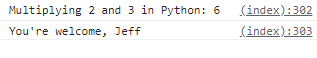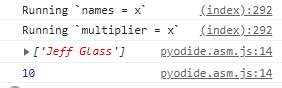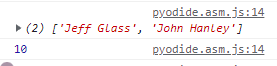# Accessing Objects Created in PyScript from JavaScript

Am I right in thinking that, because PyScript doesn’t expose its internal Pyodide instance, you can’t access objects created in a PyScript tag from JavaScript?

I know that when using Pyodide directly, one can do:

``````<script type="module">
const pyodideRuntime = await loadPyodide();
pyodideRuntime.runPython(`
# A Python variable
name = "Jeff"

# Define a Python function
def multiplyTwoNumbers(x, y):
return (x * y)
`);

// Access variable and function in JavaScript
let mult = pyodideRuntime.globals.get('multiplyTwoNumbers');
console.log("Multiplying 2 and 3 in Python: " + mult(2,3));
console.log("You're welcome, " + pyodideRuntime.globals.get('name'))
</script>
``````But without access to PyScript’s Pyodide instance, I don’t think it’s possible access Python objects created in PyScript.

Is there a workaround I’m missing?

1 Like

The Python code can call JavaScript code and pass Python objects which include functions. IIRC the JavaScript code can continue to access the Python objects and call Python functions. I need to double-check that.

1 Like

I’ve found a way of binding a JavaScript variable to a Python object created in PyScript by using Javascript’s `eval()` method. I can’t imagine this is the best way to do this, but I thought it was interesting, and maybe useful to others exploring related topics down the road.

We create a Javascript function that takes an object and a string, and uses eval() to bind that object to a new variable with that string as it’s name.

``````<script>
function createObject(x, variableName){
let execString = variableName + " = x"
console.log("Running `" + execString + "`");
eval(variableName + " = x")
}
</script>
``````

Then in PyScript, we call that function with the object’s we’d like to pass and their name

``````<py-script>
import js
from pyodide import create_proxy, to_js

names = ["Jeff Glass"]
js.createObject(create_proxy(names), "names")

multiplier = lambda z: z * 2
js.createObject(create_proxy(multiplier), "multiplier")

console.log(to_js(js.names))
console.log(js.multiplier(5))
</py-script>
``````Then if we mutate any of the Python objects, we can see the change reflected in JavaScript.

Of course the Javascript variable is bound to the Python object, not the Python variable name. Here:

• the list `names` is mutated, but
• the variable python variable `multiplier` is now points to the new lambda function
• while the Javascript variable `multiplier` still points to the original lambda function:
``````# continued from above
<py-script>
names.append("John Hanley")
console.log(to_js(js.names))

multiplier = lambda z: z * 100000
console.log(js.multiplier(5))
</py-script>
``````1 Like

I’ve written up these results into a more coherent example over on my blog, as well as the process of passing variables between JS and Python more generally; that’s referenced in a new post on this forum.. I figured it’d be nice to not continue my ramblings in this particular thread.1 Like